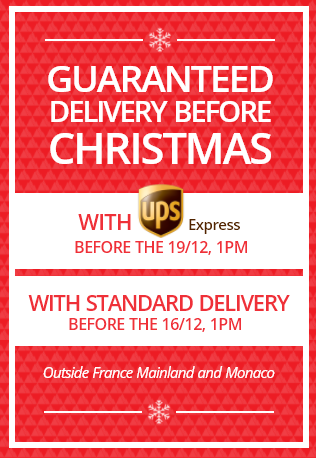100 days to change your mind

# Kids snowboards

Nowadays, brands make kids-specific lines of snowboards. Suited for their needs, these boards are playful and progressive to help young riders become shredders! There is no right age to begin snowboarding and we can add to this 'the younger, the better'. Get him a board adapted to his style and needs and watch him do stuff you never did yourself! Discover all of our kids snowboards with brands like Nitro, Lib Tech.

(=showDesc ? 'Show less' : 'Read more' =)
SANTA IS RIDING! Get an extra 15% off with the code SANTAAvailable on all products marked as "extra 15% off", just enter the code when confirming your cart.
Extra 15% off
Recommended price £165.91
-40%
Recommended price £165.91
-40%
• From From £99.52
Or (=dividePaymentUnit=)x(=priceDivided =)(=itemCurrency=)
• From From £99.52
Or (=dividePaymentUnit=)x(=priceDivided =)(=itemCurrency=)
Extra 15% off
• From From £165.91
Or (=dividePaymentUnit=)x(=priceDivided =)(=itemCurrency=)
• From From £165.91
Or (=dividePaymentUnit=)x(=priceDivided =)(=itemCurrency=)
From
Extra 15% off
• From From £165.91
Or (=dividePaymentUnit=)x(=priceDivided =)(=itemCurrency=)
• From From £165.91
Or (=dividePaymentUnit=)x(=priceDivided =)(=itemCurrency=)
Extra 15% off
• From From £141.01
Or (=dividePaymentUnit=)x(=priceDivided =)(=itemCurrency=)
• From From £141.01
Or (=dividePaymentUnit=)x(=priceDivided =)(=itemCurrency=)
From
Extra 15% off
• From From £141.01
Or (=dividePaymentUnit=)x(=priceDivided =)(=itemCurrency=)
• From From £141.01
Or (=dividePaymentUnit=)x(=priceDivided =)(=itemCurrency=)
Extra 15% off
• From From £165.91
Or (=dividePaymentUnit=)x(=priceDivided =)(=itemCurrency=)
• From From £165.91
Or (=dividePaymentUnit=)x(=priceDivided =)(=itemCurrency=)
Extra 15% off
Recommended price £165.91
-30%
Recommended price £165.91
-30%
• From From £116.11
Or (=dividePaymentUnit=)x(=priceDivided =)(=itemCurrency=)
• From From £116.11
Or (=dividePaymentUnit=)x(=priceDivided =)(=itemCurrency=)• From From £141.01
Or (=dividePaymentUnit=)x(=priceDivided =)(=itemCurrency=)
• From From £141.01
Or (=dividePaymentUnit=)x(=priceDivided =)(=itemCurrency=)
Extra 15% off
• From From £149.32
Or (=dividePaymentUnit=)x(=priceDivided =)(=itemCurrency=)
• From From £149.32
Or (=dividePaymentUnit=)x(=priceDivided =)(=itemCurrency=)
• From From £165.91
Or (=dividePaymentUnit=)x(=priceDivided =)(=itemCurrency=)
• From From £165.91
Or (=dividePaymentUnit=)x(=priceDivided =)(=itemCurrency=)
• From From £74.62
Or (=dividePaymentUnit=)x(=priceDivided =)(=itemCurrency=)
• From From £74.62
Or (=dividePaymentUnit=)x(=priceDivided =)(=itemCurrency=)
Extra 15% off
• From From £231.58
Or (=dividePaymentUnit=)x(=priceDivided =)(=itemCurrency=)
• From From £231.58
Or (=dividePaymentUnit=)x(=priceDivided =)(=itemCurrency=)
• From From £190.81
Or (=dividePaymentUnit=)x(=priceDivided =)(=itemCurrency=)
• From From £190.81
Or (=dividePaymentUnit=)x(=priceDivided =)(=itemCurrency=)
• From From £141.01
Or (=dividePaymentUnit=)x(=priceDivided =)(=itemCurrency=)
• From From £141.01
Or (=dividePaymentUnit=)x(=priceDivided =)(=itemCurrency=)
Extra 15% off
Recommended price £165.91
-30%
Recommended price £165.91
-30%
• From From £116.11
Or (=dividePaymentUnit=)x(=priceDivided =)(=itemCurrency=)
• From From £116.11
Or (=dividePaymentUnit=)x(=priceDivided =)(=itemCurrency=)
• From From £224.02
Or (=dividePaymentUnit=)x(=priceDivided =)(=itemCurrency=)
• From From £224.02
Or (=dividePaymentUnit=)x(=priceDivided =)(=itemCurrency=)
From
• From From £224.02
Or (=dividePaymentUnit=)x(=priceDivided =)(=itemCurrency=)
• From From £224.02
Or (=dividePaymentUnit=)x(=priceDivided =)(=itemCurrency=)
• From From £199.12
Or (=dividePaymentUnit=)x(=priceDivided =)(=itemCurrency=)
• From From £199.12
Or (=dividePaymentUnit=)x(=priceDivided =)(=itemCurrency=)
• From From £190.81
Or (=dividePaymentUnit=)x(=priceDivided =)(=itemCurrency=)
• From From £190.81
Or (=dividePaymentUnit=)x(=priceDivided =)(=itemCurrency=)
• From From £248.92
Or (=dividePaymentUnit=)x(=priceDivided =)(=itemCurrency=)
• From From £248.92
Or (=dividePaymentUnit=)x(=priceDivided =)(=itemCurrency=)
• From From £232.32
Or (=dividePaymentUnit=)x(=priceDivided =)(=itemCurrency=)
• From From £232.32
Or (=dividePaymentUnit=)x(=priceDivided =)(=itemCurrency=)
Extra 15% off
• From From £224.02
Or (=dividePaymentUnit=)x(=priceDivided =)(=itemCurrency=)
• From From £224.02
Or (=dividePaymentUnit=)x(=priceDivided =)(=itemCurrency=)
• From From £240.61
Or (=dividePaymentUnit=)x(=priceDivided =)(=itemCurrency=)
• From From £240.61
Or (=dividePaymentUnit=)x(=priceDivided =)(=itemCurrency=)
• From From £199.12
Or (=dividePaymentUnit=)x(=priceDivided =)(=itemCurrency=)
• From From £199.12
Or (=dividePaymentUnit=)x(=priceDivided =)(=itemCurrency=)
Extra 15% off
• From From £232.32
Or (=dividePaymentUnit=)x(=priceDivided =)(=itemCurrency=)
• From From £232.32
Or (=dividePaymentUnit=)x(=priceDivided =)(=itemCurrency=)
• From From £165.91
Or (=dividePaymentUnit=)x(=priceDivided =)(=itemCurrency=)
• From From £165.91
Or (=dividePaymentUnit=)x(=priceDivided =)(=itemCurrency=)
Extra 15% off
Recommended price £232.32
-30%
Recommended price £232.32
-30%
• From From £162.60
Or (=dividePaymentUnit=)x(=priceDivided =)(=itemCurrency=)
• From From £162.60
Or (=dividePaymentUnit=)x(=priceDivided =)(=itemCurrency=)

## Buyer’s guide for a kids snowboard

What length and what type of board does your kid need? The ideal board is be the one that’s suited to his age and to his skills, so that mini champs can have fun straight away. Here are our tips, age per age.

### What is a good snowboard for kids?

Manufacturers utilise technologies tested and proven to be efficient on adult boards which they derive to make them playful and forgiving. Kids snowboards come with a soft flex making the board easier to manage.

### What board should you buy for a 5 / 6 year old kid?

In average, a 5 or 6 year-old kid is between 3 and 4 feet tall. This means he needs a board between 80 and 115cm. The most important thing is to make things easy. Favour forgiving boards, something with an easy pivot will help kids link turns better. Flashy and fancy graphics are a bonus feature, it’s always nicer to have a board that looks nice!

### What kind of snowboard if your kid is between 7 to 9 years old?

A 7 to 9 year old kid measures between 3'5" and 4'5". His board length should fit between 100 and 130cm. Boards designed for kids of this age are really fun to ride and really playful. They are flexible and forgiving. Rockers have made them a lot easier to manoeuver and catchfree.

### Which board should you pick of your child is 10 to 12 years old?

At this age, most kids measure 4'2" up to 5'2". The matching board length is 115 up to 140cm. Pre-teens begin to have tastes and they will probably ask for specific graphics but remember, the most important thing is to get them something suited for their skills. Those who have been riding for years will want something lively and powerful while beginners will prefer something soft and easy.